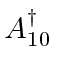### Harmonic Oscillator Raising Operator

We wish to find the matrix representing the 1D harmonic oscillator raising operator.

We use the raising operator equation for an energy eigenstate.Now simply compute the matrix element.Now this Kronecker delta puts us one off the diagonal. As we have it set up, i gives the row and j gives the column. Remember that in the Harmonic Oscillator we start counting at 0. For i=0, there is no allowed value of j so the first row is all 0. For i=1, j=0, so we have an entry forin the second row and first column. All he entries will be on a diagonal from that one.Jim Branson 2013-04-22